# 7. Bouncing Around the Screen¶

In these notes you will learn:

• How to make a ball move in any direction at a constant speed.
• Some basic uses of if-statements.
• How to perform simple actions when an edge is hit (such as stopping or expanding).

## 7.1. Introduction¶

Now lets create a program that makes a ball — or any shape or image — move in any direction and bounce off any edge of the screen.

To allow a ball to move in any direction we need to take into account its speed in both the x-direction and the y-direction. Thus we will be using two variables, dx and dy, to control the ball’s speed. The variable dx is how many pixels the ball moves in the x-direction on each call to draw(), and dy is how many pixels it moves in the y-direction.

Another assumption we will make is that every time the ball hits an edge it bounces off at an out-going angle that is the same as the incoming angle. i.e. a = b in the following diagram: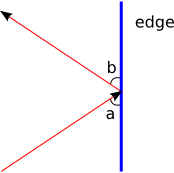Finally, we will ignore things like gravity and air friction. Such details can be added later.

## 7.2. Variables and Initialization¶

Lets walk through the creation of our bouncing-ball program step-by-step. First, we need a couple of variables to keep track of the position of the ball:

```float x;   // (x, y) is the center
float y;   // of the ball
```

And then we need two speed variables to keep track of how far the ball moves along the x-axis and y-axis on each call to draw():

```float dx;
float dy;
```

For convenience, lets also add a couple of color variables:

```color orange = color(255, 165, 0);
color white = color(255, 255, 255);
```

Note that all six of these variables are declared outside of any function, i.e. they are all global variables.

We initialize the screen and the four ball variables in setup():

```void setup() {
size(500, 500);
smooth();

// set the ball's initial position and velocity
x = 250;    // start in the center of the screen
y = 250;
dx = 1;   // move up and to the right
dy = -2;
}
```

The precise starting values of x, y, dx, and dy, are, of course, up to you.

## 7.3. Animating the Ball¶

Now we need to think about how to animate the ball. The essential idea is to repeat the following steps:

• draw the background
• draw the ball at (x, y)
• move the ball to its next position by adding dx to x and dy to y
• check if the ball has hit one of the four edges, and, if so, change the direction of the ball by negating either dx or dy

The above way of describing a program is called pseudocode: it is a little bit like computer code, but written in English. We can also describe code using a flow chart. However, flow charts are not very common with modern programmers since they take up a lot more space than pseudocode without providing much (or any) more information.Following the flow chart, the actual Processing code we write uses four if- statements, one for each edge:

```void draw() {
// re-draw the background
background(white);

// draw the ball
noStroke();
fill(orange);
ellipse(x, y, 50, 50);

// move the ball to its next position
x += dx;
y += dy;

// hit the top edge?
if (y <= 0) {
dy = -dy;
}

// hit the bottom edge?
if (y >= 499) {
dy = -dy;
}

// hit the left edge?
if (x <= 0) {
dx = -dx;
}

// hit the right edge?
if (x >= 499) {
dx = -dx;
}
}
```

## 7.4. Another Way to Write the If-statements¶

There’s often more than one correct way to write an if-statement. For instance, we can use || (“or”) to combine the top/bottom test into one statement, and the left/right test into another:

```// hit top or bottom edge?
if (y <= 0 || y >= 499) {
dy = -dy;
}

// hit left or right edge?
if (x <= 0 || x >= 499) {
dx = -dx;
}
```

The expression y <= 0 || y >= 499 is true when y <= 0 is true, or when y >= 499 is true, or when both y <= 0 and y >= 499 are true. The only time it is false is when both y <= 0 is false, and y >= 499 is false. Here it is a flow chart: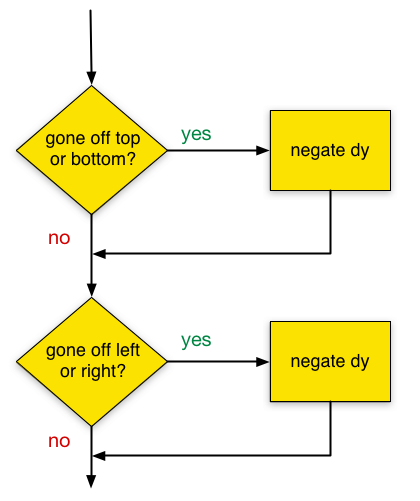It might seem that two if-statements are preferable to four — it’s less code after all!. But it’s not so simple. First, the two if-statement version has more complicated conditions. Second, the two if-statement version is less changeable. If you use this code in, say, a game, then it’s likely that you’ll want something different to happen to the ball depending on which edge it hits. Maybe the top edge is the sky and the bottom edge is the ground, and so the ball should fly off the screen if it hits the top, but bounce when it hits the bottom. You’ll need one if-statement for each different kind of edge behaviour, as we wrote in the first program.

## 7.5. Bouncing Off the Ball’s Edge¶

The basic bouncing ball program can be changed in many different ways. For instance, right now the ball changes direction when its center points hits an edge, which causes part of the ball to go off the screen.

Suppose, instead, we want the ball never to leave the screen when it bounces. Then we need to test if one of its four edge points has gone off the screen: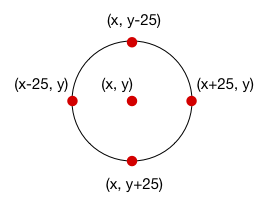Recall that, by default, Processing sets the location on an ellipse by specifying its center point, (x, y). So when the ball hits the right edge of the screen, we want it to reverse its x-direction as soon as its right-most point — (x + 25, y) — hits the right edge. Similarly, we want the ball to reverse its y-direction when the point (x, y + 25) hits the bottom edge.

Thus we can change the if-statements in draw to test for these four new points:

```// hit the top edge?
if (y - 25 <= 0) {
dy = -dy;
}

// hit the bottom edge?
if (y + 25 >= 499) {
dy = -dy;
}

// hit the left edge?
if (x - 25 <= 0) {
dx = -dx;
}

// hit the right edge?
if (x + 25 >= 499) {
dx = -dx;
}
```

Make these changes in the program and run it. You’ll see that it makes the ball and screen-edges seem solid.

## 7.6. Stopping at the Top¶

Lets set ourselves another problem: how can we make the ball stop moving completely when it hits the top of the screen?

It turns out to be a simple change:

```// hit the top edge?
if (y - 25 <= 0) {
dx = 0;
dy = 0;
}
```

We modify the code in the body of the if-statement that checks for hitting the top edge to set the speed of the ball to be 0 in both the x-direction and y-direction. This cases the ball to stop dead as soon as it hits the top edge of the screen.

Keep in mind that even though the ball is not moving, draw() is still being called. The ellipse is still being drawn, and x and y are still being updated. But its position never changes because dx and dy are both 0.

## 7.7. Expanding When it Hits the Top¶

How can we make the ball bigger when it hits the top of the screen? In other words, we want the diameter of the ball to get bigger every time hits the top edge.

Currently, the diameter of the ball is always set to 50:

```ellipse(x, y, 50, 50);
```

Since our diameter is now going to change, we need to replace 50 with a variable that stores the current diameter. So lets add the variable diam:

```// ... variables as before ...

float diam;

void setup() {
// ... code as before ...
diam = 50;
}

void draw() {
// ... code as before ...

// draw the ball
noStroke();
fill(orange);
ellipse(x, y, diam, diam);

// ... code as before ...
}
```

Now that the diameter of the ball is a variable, we can change it:

```// hit the top edge?
if (y - 25 <= 0) {
dy = -dy;
diam += 10;
}
```

Make this change and run the program, and you should see that the ball gets a little bit bigger each time it hits the top edge.

A problem you might notice is that part of the ball now goes off the edge of the screen. We didn’t have this problem when the diameter was fixed. What is going on?

The problem is caused by the if-statement conditions that check for edge collisions: they all assume that the diameter of the ball is 50. For example:

```// hit the right edge?
if (x + 25 >= 499) {
dx = -dx;
}
```

The condition here is x + 25 >= 499, and we use x + 25 because that’s the x-coordinate of the right-most point of the circle of diameter 50. But the diameter of our circle is not 50: it’s diam. So we have to re-write the condition like this:

```// hit the right edge?
if (x + diam / 2 >= 499) {
dx = -dx;
}
```

The expression x + diam / 2 is the x-value of the right-most point of a ball of diameter diam: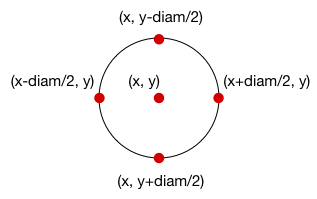So we need to adjust each if-statement to take diam into account:

```// hit the top edge?
if (y - diam / 2 <= 0) {
dy = -dy;
diam += 10;
}

// hit the bottom edge?
if (y + diam / 2 >= 499) {
dy = -dy;
}

// hit the left edge?
if (x - diam / 2 <= 0) {
dx = -dx;
}

// hit the right edge?
if (x + diam / 2 >= 499) {
dx = -dx;
}
```

Make these changes to the program and see what happens. Does it work?

## 7.8. Debugging: Finding a Bug¶

Unfortunately, the above changes don’t quite work. When I run the modified program the ball gets stuck on the top edge of the screen and inflates to a huge size without ever bouncing off any other edges. Obviously something is wrong with the program. But what?

This is a tricky error to deal with because there is no obvious flaw in any line of our code. The fact that the problem only occurs when the ball hits the top edge of the screen gives a hint about where the problem might be:

```// hit the top edge?
if (y - diam / 2 <= 0) {
dy = -dy;
diam += 10;
}
```

To figure out what is going on, it is helpful to know what lines of code are being called. A simple way to check this is to use a println statement to print a message every time the code in the if-statement body is called:

```// hit the top edge?
if (y - diam / 2 <= 0) {
println("hit top edge?");
dy = -dy;
diam += 10;
}
```

println prints a message in the black window at the bottom of the Processing editor know as the console window. We usually use println to help us understand and fix programs.

When you run the code after inserting this println, the ball still gets stuck and inflates at the top of the screen. But you should now see this in the black window at the bottom:

```hit top edge?
hit top edge?
hit top edge?
hit top edge?
...
```

What this tells us is that the body of the top-edge if-statement is being called again and again. Somehow, the top point of the ball has gotten stuck above the top edge of the screen and can’t get out.

How might this happen? Lets try one more little trick: lets print the values of y, dy, and diam the first time the top-edge if-statement body is called, and then lets immediately stop the program using System.exit(0) to see the results:

```if (y - diam / 2 <= 0) {
println("hit top edge?");
println("diam = " + diam);
println("diam / 2 = " + diam / 2);
println("y = " + y);
System.exit(0);  // immediately stops the program

dy = -dy;
diam += 10;
}
```

Here’s the output that is produced on the console when the program runs:

```hit top edge?
diam = 50.0
diam / 2 = 25.0
y = 22.0
dy = -4.0
```

This tells us the value of all the relevant variables the first time that y - diam / 2 <= 0 evaluates to true. The expression y - diam / 2 is less than 0 because y is 22 and diam / 2 is 25.

So that makes sense. Now lets imagine what happens when the next two statements are run:

```dy = -dy;
diam += 10;
```

After running these, dy is 4, diam is 60, and y is still 22. This is where the problem lies: the expression y - diam / 2 <= 0 is true because if you substitute the values for their variables, you get the true expression 22 - 30 <= 0. So the point we use to test if the ball has gone off the top edge of the screen is already off the top edge!

The next time draw() is called, y gets incremented by 2 (by y += dy), and so is now 24. Thus y - diam / 2 <= 0 is true (because it is 24 - 60 / 2 <= 0), and the top-edge if-statement body is executed once more. When that runs (ignoring System.exit(0) now), diam becomes 70 and dy becomes -2. The next time draw() is called, y is back to 22 and diam is 70, and so y - diam / 2 <= 0 is still true.

So y flips back and forth between 24 and 26 on each call to draw(). Since diam also increases on each call, the expression y - diam / 2 <= 0 remains true. Thus the ball is trapped off the top edge of the screen.

This is a tricky problem to catch. To understand it, you need to trace through a few calls to draw() to see that the values of y are stuck at 24 and 26. These sorts of errors get easier to catch with experience, but you can never be rid of them completely: learning strategies for finding and fixing subtle bugs is an important part of programming.

## 7.9. Debugging: Fixing the Problem¶

So we’ve identified the problem, but how do we fix it? This is not completely obvious because there are many ways that we could modify the value of y (or even dy) that might fix it.

But lets try to understand a little more what is going on. The first time that the top-edge if-statement condition is true, the image on the screen looks something like this: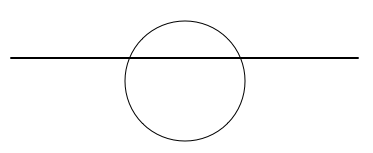The top of the ball goes a little bit into the top edge of the screen, i.e. the ball interpenetrates the edge. The ball and the edge are supposed to be hard surfaces that don’t allow any sort of interpenetration. But our arithmetic allows for them to intersect, and so we have to do something to stop that.

The solution we’ll use is as follows: when the ball interpenetrates an edge, we’ll rest its position to be just barely touching the edge. For example:

```// hit the left edge?
if (x - diam / 2 <= 0) {
dx = -dx;
x = diam / 2;
}
```

In this if-statement, when the ball hits the left edge of the screen we reverse its direction, and then set x so that the ball is just touching the edge without going over. In a sense, we are re-setting the position of the ball every time it hits an edge.

We have to reset the ball’s position for each if-statement:

```// hit the top edge?
if (y - diam / 2 <= 0) {
dy = -dy;
diam += 10;
y = diam / 2;
}

// hit the bottom edge?
if (y + diam / 2 >= 499) {
dy = -dy;
y = 499 - diam / 2;
}

// hit the left edge?
if (x - diam / 2 <= 0) {
dx = -dx;
x = diam / 2;
}

// hit the right edge?
if (x + diam / 2 >= 499) {
dx = -dx;
x = 499 - diam / 2;
}
```

This works! Run it and you will see that the ball now stays on the screen and expands when it hits the top.

## 7.10. Questions¶

1. What is pseudocode?

2. Pseudocode and flow charts can both be used to represent programs. List the pros and cons of each.

3. For each of the following expressions, state if it is true or false. Assume that x, y, dx, and dy are defined as follows:

```float x = 32;
float y = -4;
float dx = 1.22;
float dy = 1.57;
```
1. x == x
2. x == y
3. dx < dy
4. (dy > dx) || (dy < dy)
5. (y == -4) || (x > 0)
6. (y < -4) || (x <= 0)
7. (x == y) || (dx == dy) || (x + y == dx + dy)
8. (1 <= 2) || (x > x)
9. (x < 0) || (x > 499) || (y < 0) || (y > 499)
10. (x > mouseX) || (y > mouseY)
4. Draw a flow chart for the following code snippet:

```if (y <= 0) {
dy = -dy;
} else if (y >= 499) {
dy = -dy;
} else if (x <= 0) {
dx = -dx;
} else if (x >= 499) {
dx = -dx;
}
```

This code is the same as the if-statements in the sample program, except the second, third, and fourth statements begin with else.

5. What does the println statement do?

## 7.11. Programming Questions¶

1. Modify the bouncing ball program so that a small ellipse is drawn under the ball as a shadow to give the illusion of 3-dimensions. For example:Make sure the shadow gets bigger as the ball’s size increases.

2. Modify the bouncing ball program so that when the ball hits the left edge of the screen, its speed increases by a factor of 1.5.

Also, keep the ball’s size fixed: do not have it change size when it hits an edge.

3. Write a program that makes a square bounce around the screen. When the squares hits an edge it should hit the edge exactly, without any part of it going off the screen.

Keep the dimensions of the square the same throughout the program (e.g. don’t let it get bigger when it hits an edge).

4. Write a program that makes an image (i.e. a PImage) bounce around the screen. When the image hits an edge it should hit the edge exactly, without any part of it going off the screen.

5. Modify the bouncing ball program so that the ball changes to red when it hits the top edge; to green when it hits the right edge; to blue when it hits the bottom edge; and to orange when it hits the left edge.

Also, keep the ball’s size fixed: do not have it change size when it hits an edge.

6. Draw a 200-by-200 square centered in the middle of a 500-by-500 screen. Write a program that makes a ball bounce inside this square.

7. Make two different colored balls bounce around the screen. You’ll need to keep track of the position, velocity, and color of each ball, so your program will need at least 10 different variables.

8. Modify the bouncing ball program so that the ball’s diameter does not change when it hits an edge. Instead, use the map function to make the balls diameter go from 25 to 150 as the mouse pointer moves horizontally across the screen.

For example, when the mouse pointer is halfway across the screen, the ball should have a diameter of about 87 (i.e. halfway between 25 and 150). When the mouse pointer is at the very left of the screen, the ball’s diameter should be 25.

9. Modify the bouncing ball program so that every fifth time the ball hits any edge it changes color. For example, it can start out orange, and the after 5 edge hits it turns red. Then after 5 more edge hits it turns red, and so on.

Also, keep the ball’s size fixed: do not have it change size when it hits an edge.

## 7.12. Code: The Bouncing Ball Program¶

This program makes a ball bounce around the screen, increasing its diameter every time it hits the top edge:

```color white = color(255);
color orange = color(255, 165, 0);

float x;
float y;
float dx;
float dy;
float diam;

void setup() {
size(500, 500);
smooth();
x = 100;
y = 100;
dx = 1;
dy = 2;
diam = 50;
}

void draw() {
background(white);

noStroke();
fill(orange);
ellipse(x, y, diam, diam);

x += dx;
y += dy;

// hit the left edge?
if (x - diam / 2 <= 0) {
dx = -dx;
x = diam / 2;
}

// hit the right edge?
if (x + diam / 2 >= 499) {
dx = -dx;
x = 499 - diam / 2;
}

// hit the top edge?
if (y - diam / 2 <= 0) {
dy = -dy;
diam += 10;
y = diam / 2;
}

// hit the bottom edge?
if (y + diam / 2 >= 499) {
dy = -dy;
y = 499 - diam / 2;
}
}
```